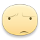Quang Nhân 9 phút trước

1. There are varieties ways of solving the problems.

2. My family live in a northern moutainous region.

3.The country's natural resources include forests, coal and oil .

4. Compost is a wonderful fertilizer.

5. How careless  of you to break that cup .

6. English is wided used in many parts of the world.

Bình luận (1)L.Ai 16 phút trước

ý bạn là gì mình ko hiểuBình luận (0)Quang Nhân 1 phút trước

My favorite TV program is Hello Jadoo - a famous program. I like this program because it is very funny and entertaining. It's on Disney Channel at 5pm from Tuesday to Saturday every week. I always watch it . This program is about a cute girl, funny and intelligent but quite lazy and friends good-hearted, genial of jadoo. Through cartoon, I developed communication skills, perseverance and life skills. I wish cartoon will premiere viewers or more episodes and more meaningful.

Bình luận (0)Nguyễn Huy Mạnh 16 phút trước

$n_{CO_2} = 0,4\ mol ; n_{H_2O} = 0,7\ mol ; n_{N_2} = 3,1\ mol$

BTNT với O :

$2n_{O_2} = 2n_{CO_2} + n_{H_2O}\\ \Rightarrow n_{O_2} = 0,75\ mol$

$\Rightarrow n_{không\ khí} = \dfrac{0,75}{20\%} = 3.75(mol) \\ n_{N_2(trong\ không\ khí)} = 3,75 - 0,75 = 3(mol)$

BTNT với C,H và N :

$n_C = n_{CO_2} = 0,4\ mol\\ n_H = 2n_{H_2O} = 1,4\ mol\\ n_N = 2n_{N_2} - 2n_{N_2\ trong\ không\ khí}= 0,2\ mol$

$n_C : n_H : n_N = 0,4 : 1,4 : 0,2 = 2 :7 :1$

Vậy CTHH của X : C2H7N

Bình luận (0)Quang Nhân 16 phút trước

$n_{CO_2}=\dfrac{17.6}{44}=0.4\left(mol\right)\Rightarrow n_C=0.4\left(mol\right)$

$n_{H_2O}=\dfrac{12.6}{18}=0.7\left(mol\right)\Rightarrow n_H=1.4\left(mol\right)$

$n_{N_2}=\dfrac{69.44}{22.4}=3.1\left(mol\right)\Rightarrow n_N=6.2\left(mol\right)$

$BtO:2n_{O_2}=2n_{CO_2}+n_{H_2O}\\ \Leftrightarrow n_{O_2}=\dfrac{2\cdot0.4+0.7}{2}=0.75\left(mol\right)$

$n_{N_{2\left(kk\right)}}=4n_{O_2}=3\left(mol\right)$

$\Rightarrow n_{N_2\left(sp\right)}=3.1-3=0.1\left(mol\right)$

$Đặt:CTHH:C_xH_yN_z$

$x:y:z=0.4:1.4:0.2=2:7:1$

$X:C_3H_7N$

Bình luận (0)Nguyễn Huy Mạnh 25 phút trước

$n_{CO_2} = 0,1 ; n_{H_2O} = 0,1$

$M_X = \dfrac{1}{ \dfrac{0,373}{22,4}} = 60(đvC)$

Bảo toàn khối lượng :

$m_{O_2} = 0,1.44 + 1,8 -3 = 3,2(gam)\\ \Rightarrow n_{O_2} = 0,1(mol)$

Bảo toàn nguyên tố với C,H và O :

$n_C = n_{CO_2} = 0,1\ mol\\ n_H = 2n_{H_2O} = 0,1.2 = 0,2\ mol\\ n_O = 2n_{CO_2} + n_{H_2O} -2n_{O_2} = 0,1(mol)\\ n_X = \dfrac{3}{60} = 0,05(mol)$

Ta có :

Số nguyên tử Cacbon = $\dfrac{0,1}{0,05} = 2$

Số nguyên tử Hidro = $\dfrac{0,2}{0,05} = 4$

Số nguyên tử Oxi = $\dfrac{0,1}{0,05} = 2$

Vậy CTPT của X : C2H4O2

Bình luận (0)🍀🧡_Trang_🧡🍀 CTV 30 phút trước

Nobody gets ..preferential..treatment in this office.  (prefer)

Bình luận (0)